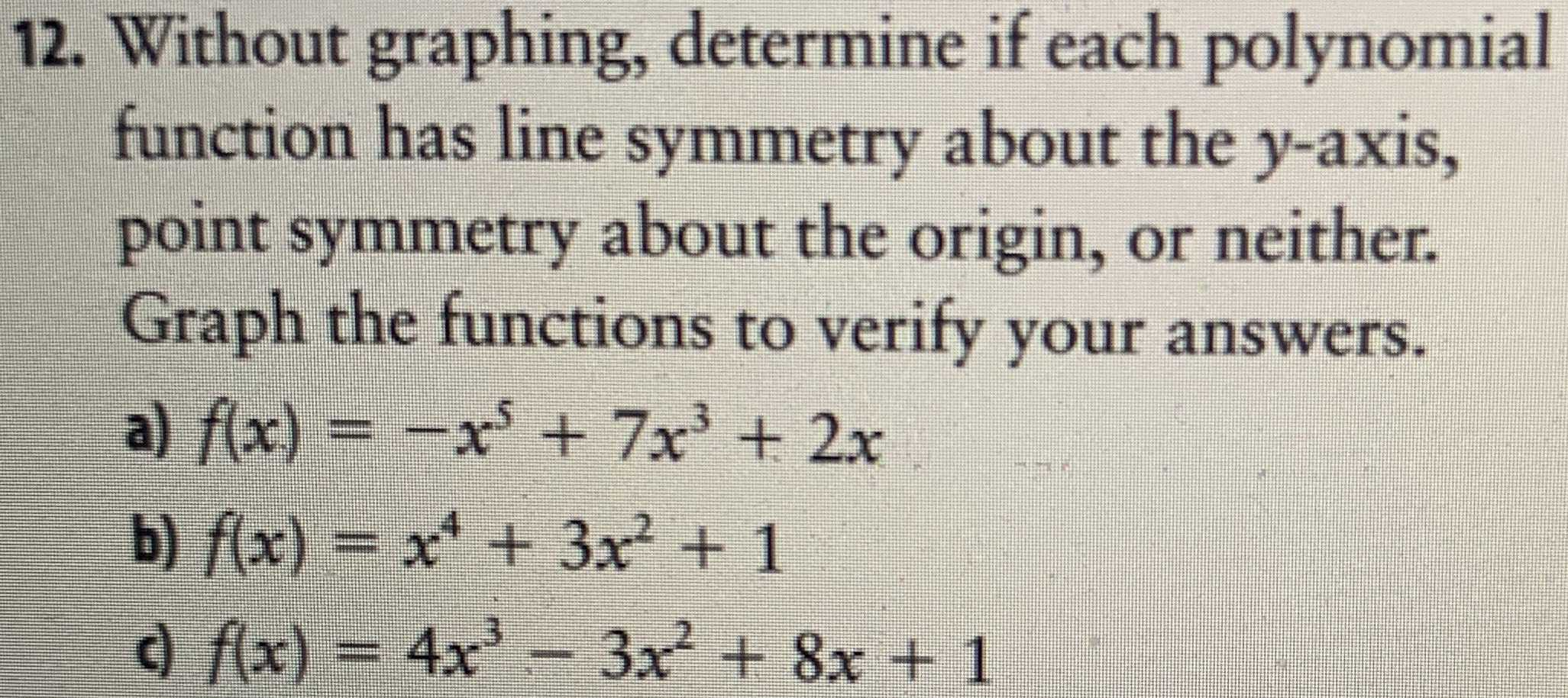### ¿Todavía tienes preguntas de matemáticas?

Pregunte a nuestros tutores expertos
Algebra
Pregunta12. Without graphing, determine if each polynomial function has line symmetry about the $$y$$ -axis, point symmetry about the origin, or neither. Graph the functions to verify your answers. a) $$f ( x ) = - x ^ { 5 } + 7 x ^ { 3 } + 2 x$$

b) $$f ( x ) = x ^ { 4 } + 3 x ^ { 2 } + 1$$

c) $$f ( x ) = 4 x ^ { 3 } - 3 x ^ { 2 } + 8 x + 1$$

a)

symmetric to origin

b)

symmetric to y - axis

c)

symmetric to ( 0, 1 )

Solución
View full explanation on CameraMath App.